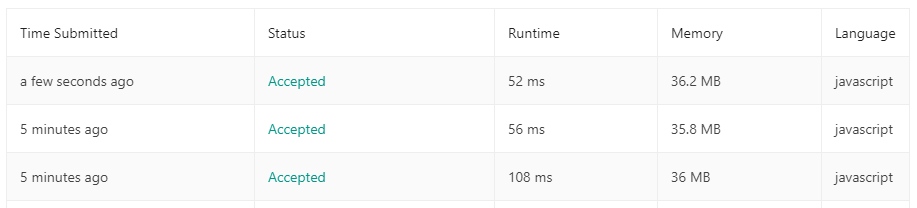#DAY 12
3
Software Development

## 1. Two Sum

``````/*
Given an array of integers, return indices of the two numbers such that they add up to a specific target.

You may assume that each input would have exactly one solution, and you may not use the same element twice.

Example:

Given nums = [2, 7, 11, 15], target = 9,

Because nums + nums = 2 + 7 = 9,
return [0, 1]
*/

/**
* @param {number[]} nums
* @param {number} target
* @return {number[]}
*/

var twoSum = function(nums, target) {

};
``````

### Think

#### 極限值/特殊狀況

• 負數
• 可能會有同樣的值
• nums.length < 2，根本無法相加。(但題目說一定會有結果所以這個可以忽略)

#### 哪種資料結構解

• 第一直覺會想到 Array

#### 大概會怎麼解

• i = 0 時， i = 2, 所以我們要找的就是 9 - 2，所以我想要 indexOf( 9 - 2)，看看有沒有。
• 都找不到的話 i++，再重覆以上

#### 用程式實踐

``````var twoSum = function(nums, target) {
const len = nums.length;
for(let i = 0; i< len; i++ ){
var ind = nums.indexOf(target - nums[i]);
if(ind !== -1 && i !== ind){
return [i, ind];
}
}

};
``````

## 再想想看有沒有更好作法

#### 哪種資料結構解

• 我們其實是想要做 "Search" 所以 Map 會是更好做法。因為 Map 在尋找跟增加效能都比 Array 好

#### 用程式實踐

``````var twoSum = function(nums, target) {
const m = new Map();
let result;
nums.forEach( (item, index) => {
let indValue = target - item;
if (m.has(indValue)) {
result = [m.get(indValue), index];
}
m.set(item, index);
})
return result;
};
``````

Runtime: 52 ms, faster than 92.70% of JavaScript online submissions for Two Sum.### 1 則留言

1``````var twoSum = function(nums, target) {
const map = {}
let result
nums.forEach((item, index) => {
let indValue = target - item;
if(typeof map[indValue] !== 'undefined') {
result = [map[indValue], index]
}
map[item] = index
})
return result
}
``````